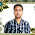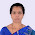Sample Questions, Previous Year Solved Papers, Study Materials For Competitive Examinations Like UGC NET, SET And GATE Computer Science.

Saturday, 25 November 2017

1.       In 8085 microprocessor which of the following flag(s) is (are) affected by an arithmetic operation?
(1) AC flag Only
(2) CY flag Only
(3) Z flag Only
(4) AC, CY, Z flags
2.       In 8085 microprocessor the address bus is of .................... bits.
(1) 4
(2) 8
(3) 16
(4) 32
3.       In the architecture of 8085 microprocessor match the following:
List - I
(a) Processing unit
(b) Instruction unit
(c) Storage and Interface unit
List - II
(i) Interrupt
(ii) General purpose Register
(iii) ALU
(iv) Timing and Control
Code :
(a)  (b)   (c)
(1) (iv) (i)    (ii)
(2) (iii) (iv)  (ii)
(3) (ii)  (iii)  (i)
(4) (i)   (ii)  (iv)
4.       Which of the following addressing mode is best suited to access elements of an array of contiguous memory locations?
(1) Indexed addressing mode
(2) Base Register addressing mode
(3) Relative address mode
(4) Displacement mode
5.       Which of the following is correct statement?
(1) In memory - mapped I/O, the CPU can manipulate I/O data residing in interface registers that are not used to manipulate memory words.
(2) The isolated I/O method isolates memory and I/O addresses so that memory address range is not affected by interface address assignment.
(3) In asynchronous serial transfer of data the two units share a common clock.
(4) In synchronous serial transmission of data the two units have different clocks.
6.       A micro-instruction format has micro-ops field which is divided into three subfields F1, F2, F3 each having seven distinct micro-operations, condition field CD for four status bits, branch field BR having four options used in conjunction with address field ADF. The address space is of 128 memory locations. The size of micro-instruction is:
(1) 17 bits
(2) 20 bits
(3) 24 bits
(4) 32 bits
7.       Consider the following four schedules due to three transactions (indicated by the subscript) using read and write on a data item X, denoted by r(X) and w(X) respectively. Which one of them is conflict serializable?
S1 : r1(X); r2(X); w1(X); r3(X); w2(X)
S2 : r2(X); r1(X); w2(X); r3(X); w1(X)
S3 : r3(X); r2(X); r1(X); w2(X); w1(X)
S4 : r2(X); w2(X); r3(X); r1(X); w1(X)
(1) S1
(2) S2
(3) S3
(4) S4
8.       Suppose a database schedule S involves transactions T1, T2, .............,Tn. Consider the precedence graph of S with vertices representing the transactions and edges representing the conflicts. If S is serializable, which one of the following orderings of the vertices of the precedence graph is guaranteed to yield a serial schedule?
(1) Topological order
(2) Depth - first order
(3) Breadth - first order
(4) Ascending order of transaction indices
9.       If every non-key attribute is functionally dependent on the primary key, then the relation is in ....................
(1) First normal form
(2) Second normal form
(3) Third normal form
(4) Fourth normal form
10.    Consider a relation R (A, B, C, D, E, F, G, H), where each attribute is atomic, and following functional dependencies exist.
CH → G
A → BC
B → CFH
E → A
F → EG
The relation R is ....................
(1) in 1NF but not in 2NF
(2) in 2NF but not in 3NF
(3) in 3NF but not in BCNF
(4) in BCNF

1.Please answer with explain of some questions

2.If every non-key attribute is functionally dependent on the primary key, then the relation is in ....................
(1) First normal form
(2) Second normal form
(3) Third normal form
(4) Fourth normal form

1.3.